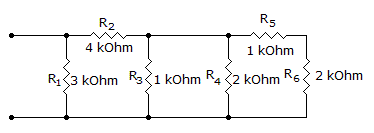# Electronics - Series-Parallel Circuits

### Exercise :: Series-Parallel Circuits - General Questions

26.

Components or combinations of components with common currents, in a series–parallel circuit, are in:

 A. parallel with each other B. series with each other C. either series or parallel with each other D. none of the above

Explanation:

No answer description available for this question. Let us discuss.

27.

What determines if resistor connections are in series, parallel, or series–parallel?

 A. the voltage source B. the power source C. resistance D. current flow

Explanation:

No answer description available for this question. Let us discuss.

28.

What is the total resistance?A. 800B. 1.2 kC. 1.6 kD. 1.8 kExplanation:

No answer description available for this question. Let us discuss.

29.

If R1 is in series with a parallel combination of R2, R3, and R4, when the resistance value of R2 increases, the voltage across R3 will

 A. increase B. decrease C. remain the same D. Cannot tell

Explanation:

No answer description available for this question. Let us discuss.

30.

A Wheatstone bridge can be used to determine an unknown

 A. current B. resistance C. power D. voltage